# Length - 6th grade (11y) - math problems

#### Number of problems found: 409

• AlishaAlisha needs 7 1/2 yards of fabric to make a quilt. She has one piece of fabric that is 1 1/2 yards and another piece of fabric that is 3 1/4 yards. How many more yards of fabric does she need to make a quilt?
• A PVC pipeA PVC pipe 12 feet long is to be cut into 1 1/2 foot pieces.  How many pieces can a plumber get out of this length pipe?
• ZarkaZarka purchased 3 1/2 meters of cloth for 100 3/8 euros. How much does 1 meter of cloth cost?
• The rope 2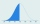The rope in the school gym is 10 ½ feet long. To make it easier to climb, Coach Hill tied a knot in the rope every ¾ foot. How many knots are in the rope?
• A rectangular 4A rectangular garden has a length of 57 m and a width of 42 m. Calculate of how many m2 will decrease the area of a garden, if the ornamental fence with a width of 60 cm will be planted inside its perimeter.
• Penny coinsOne 2p coin is 1/8 inch thick. A pile of 2p is 1 1/2 inch high. How many coin are there in the pile
• The length 7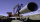The length of a water pipe is 7 m. How many ¼ m length can Jane cut from the pipe?
• A piece 3A piece of rectangle cloth has an area of 2 4/5 meters. Its breadth is 1 1/5 meters what is the length of the cloth?
• The length 6The length of 12 pipes is 10 1/2 meters. (1) find the length of one pipe (2) also find the length of 7 pipes
• The diagonals 2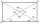The diagonals AC and BD of a rectangle ABCD intersect each other at a point O. If OA=4cm, find AC and BD.
• Backyard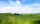Corey's backyard is rectangular with a length of 23 miles. If the area of the backyard is 12 square miles, what is its width?
• The length 5The length and breadth of rectangular field is 3 1/5 m  and 1 3/7 m. Find the area of rectangular field.
• Three piecesHana has 3 pieces of sticks measuring 0.365m, 0.152m and 0.537 respectively is the stick if she put them end to end?
• Joy has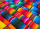Joy has 3/4 yards of fabric. She cuts the fabric into pieces that are each 1/8 yard long. How many pieces of fabric does Joy have?
• Find areaFind area of a rectangle with a length of 58 foot and a width of 49 foot?
• A plane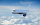A plane is flying at the rate of 350 mph after an eight hour flight is at its destination how far did the plane fly?
• Each ofEach of Suzan's notebooks is 4/5  inches wide. If she has 25 inches of space remaining on her bookshelf, how many notebooks will fit?
• A rectangular 3A rectangular plot of land has an area of 3/2 square kilometers and a length of ¾ kilometer.  What is the width of the plot of land?
• Rope cuttingShane has a piece of rope that is 7 4/5 units long. If he cuts it into pieces that are each 3/5 of a unit long, how many pieces does he have?
• BookshelfBill is cutting boards to build a bookshelf for his apartment. While cutting boards he accidently cut 3/16th ft. His board is 3 3/4th, how long should it have been?

Do you have an exciting math question or word problem that you can't solve? Ask a question or post a math problem, and we can try to solve it.

We will send a solution to your e-mail address. Solved examples are also published here. Please enter the e-mail correctly and check whether you don't have a full mailbox.

Do you want to convert length units? Length - math problems. Examples for 6th grade (the sixth graders).